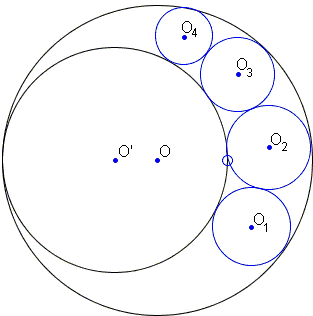# 1 + 27 = 12 + 16 Sangaku

The sangaku problem below is just another simple one that deals with chains of inscribed circles. It's included in the collection by Fukagawa and Pedoe (1.7.2), who found it not on a tablet but in a 1810 book.The circle O'(r') touches O(r) internally, and a chain of contact circles Oi(ri), i = 1, 2, 3, 4, is inscribed in the lune formed by O(r) and O'(r'). Show that

1 / r1 + 3 / r3 = 3 / r2 + 1 / r4.

SolutionThe sangaku is easily solved by a general formula for the radius of the circles in the lune:

rt = r r'(r - r') / (r r' + t²(r - r')²),

where circles in the chain tangent to each other correspond to values of t different by 1! Using A = r r' and B = r - r', we can then write

r1 = A / (A + B²),
r2 = A / (A + 2²B²),
r3 = A / (A + 3²B²),
r4 = A / (A + 4²B²).

Substituting this into

 1 / r1 + 3 / r3 = 3 / r2 + 1 / r4.

we get to verify that

 1 + 3·3² = 3·2² + 4²,

which is of course true.## Sangaku